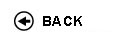Politecnico di Torino
Academic Year 2009/10
02AJRDR
Mechanics of materials
1st degree and Bachelor-level of the Bologna process in Mechanical Engineering - Vercelli
 Teacher Status SSD Les Ex Lab Tut Years teaching Soma' AurelioPO ING-IND/14 50 0 12 0 11
 SSD CFU Activities Area context ING-IND/14 5 B - Caratterizzanti Ingegneria meccanica
 Objectives of the course The course presents the basis of the mechanics of elastic bodies. Main goal is to present the fundamentals and basic knowledge for the structural design and calculus of the machine components and the mechanics of solids. Equilibrium, compatibility and constitutive equations , stress and strain relation are presented for three-dimensional bodies and the one-dimensional bodies. Expected skills The course presents the basis of the mechanics of elastic bodies. Main goal is to present the fundamentals and basic knowledge for the structural design and calculus of the machine components and the mechanics of solids. Prerequisites The student must have frequented the courses of 'Calculus' , 'Technical drawing', 'Materials engineering' Syllabus Static ' Introduction to the practical aspects of machine design is analyzed in order to understand engineering assumptions and solution idealizations. Equilibrium equations. Forces, moments, forces systems. Classification of constraints, static and hyper statically systems.. Calculus of reaction forces and moments for simple structure. Introduction to Continuum mechanics ' Stress tensor: normal and tangential stress, principal direction, Mohr circles. Strain tensor: principal direction. Constitutive laws , relation of elasticity between stress and strain. Material Strength ' Tensile test: experimental determinations and parameters. Ideal stress and strength criteria. Failure criterion. Safety coefficient for static design. Geometric properties of plane areas De Saint Venant's problem; hypotheses, cantered axial loading, bending, eccentric axial loading; torsion (circular and general cross section), shear. Deflection of beams: second order differential equation. Symmetry; force method; displacement method; continuous beams; framed structures. Stability of elastic equilibrium of rods Laboratories and/or exercises Example problems will be solved to help students understand how to use our analytical knowledge to solve practical problems. For failure criterion and beam deflection practical lab has been done during the course. Each student has to prepare a short work about the practical lab results. Bibliography Textbook: A. Somŕ Appunti del corso. R.C. Juvinall, K.M. Marshek, 'Fondamenti della Progettazione dei Componenti delle Macchine', Edizioni ETS. Revisions / Exam Exams consist in two parts: written and oral: Written examination consists in the solution of one or two exercises regarding simple design problems, as developed in the practical lab. No books or student notes are admitted. In the oral examination the practical lab will be discussed. Programma definitivo per l'A.A.2009/10© Politecnico di Torino
Corso Duca degli Abruzzi, 24 - 10129 Torino, ITALY